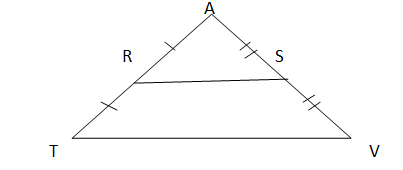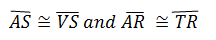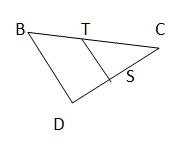# Midpoint Theorem: Definition & Application

An error occurred trying to load this video.

Try refreshing the page, or contact customer support.

Coming up next: Perpendicular Bisector: Definition, Theorem & Equation

### You're on a roll. Keep up the good work!

Replay
Your next lesson will play in 10 seconds
• 0:01 What Is the Midpoint Theorem?
• 1:46 Theorem Application
• 3:55 Lesson Summary

Want to watch this again later?

Timeline
Autoplay
Autoplay
Speed

#### Recommended Lessons and Courses for You

Lesson Transcript
Instructor: Ellen Manchester
Postulates and proven theorems allow us to know and understand certain things about geometric figures. In this lesson, we will be learning about the Midpoint Theorem.

## What is the Midpoint Theorem?

A midpoint is a point on a line segment equally distant from the two endpoints. The Midpoint Theorem is used to make a bold statement regarding triangle sides and their lengths. Given a triangle, if we connect two sides with a line segment, and this line segment joins each of the two sides at the centers, or midpoints of each side, we can know two very important aspects about the triangle and the relationships between the sides.

The Midpoint Theorem states that the segment joining two sides of a triangle at the midpoints of those sides is parallel to the third side and is half the length of the third side.

Anytime you have a line segment that connects two sides of a triangle at the midpoints, you automatically know that the sides are cut in half, and that the segment is parallel to the third side of the triangle. Parallel sides are shown by using this symbol ||. You also know the line segment is one-half the length of the third side.

Take a look at this figure:This indicates that points R and S are midpoints of sides AT and AV, respectively. From the Midpoint Theorem, since the segment RS connects the two sides at the midpoints, then RS || TV and RS is one-half the length of side TV.

This theorem allows us to prove some things about the triangle. First, if we know the length of TV, then we can figure out the length of RS, and vice-versa, since RS = ½(TV). It also allows us to find the lengths of AS, VS, TR and AR. Since RS is parallel to TV, then we also know the distance between these two line segments are equal.

## Application of the Midpoint Theorem

Let's see what we can find out about triangle BCD and each of its sides. Given that line segment TS connects the two sides BC and DC at the respective midpoints, T and S.

• If BD = 26 cm, find the length of segment TS.
• If BT = 15 cm, what is the length of segment CT?
• If CD = 20 cm, what are the lengths of both segments SD and CS?To unlock this lesson you must be a Study.com Member.

### Register to view this lesson

Are you a student or a teacher?

### Unlock Your Education

#### See for yourself why 30 million people use Study.com

##### Become a Study.com member and start learning now.
Back
What teachers are saying about Study.com

### Earning College Credit

Did you know… We have over 160 college courses that prepare you to earn credit by exam that is accepted by over 1,500 colleges and universities. You can test out of the first two years of college and save thousands off your degree. Anyone can earn credit-by-exam regardless of age or education level.